## Wednesday, July 16, 2008

### Simple Lisp Implementation in Java (Ode to the Linked List)

Overview
I have never had much affinity for writing (in natural language); I have grown up and enjoy programming and that is what I continue to do 20 years later. But I really wanted to get this blog entry out and it helped me rethink and reframe how I look at a particular piece of code. So I am going to move in all different directions starting with one of the most elementary data structures in Computer Science, the linked list. Instead of thinking of Lisp code (for now anyway) as a "listing" of elements. Think of Lisp code as a linked list of elements.
• How do you implement a linked list in C (or Java or Fortran)?
• How would you print all of the elements of the linked list?
• How would you sum all of the elements?
After thinking about the last numeric operation? Can you think of possible ways to implement various List operations, Lisp operations like 'map'?

Why are linked lists important to Lisp? "The name Lisp derives from "List Processing Language". Linked lists are one of Lisp languages' major data structures"

In a Java/C/C++ or other procedural language program, the linked list is normally composed of two fields, the data itself (composed of any Object type) and a "pointer" or reference to the next element A list that is linked. In the Java code snippet below, data refers to the element at the current node in the list. The Node object "next" is a reference to the next node in the list chain.

`package org.bot.jscheme.linkedlist;/** * A Node has two fields, data and next (or car and cdr). * This forms the basis of a simple, singly-linked list structure  * whose contents can be manipulated with cons, car, and cdr.  */public class Node {    /**     * The first element of the pair.     */    public Object data;    /**     *  The other element of the pair.     */    public Node next;    /** Build a pair from two components. * */    public Node(Object data, Node next) {        this.data = data;        this.next = next;    }    /**     * Return a String representation of the pair.     */    public String toString() {        return "" + data;    }}`

The linked list Java class is also as simple as the Node class. There is one field "head" which is a Node type. The head is the first Node in the list chain. The insert at head operation inserts a newly created Node object. It replaces the head Node with the new node and the head's next node becomes the previous node. For example, the code below contains a complete implementation.
`package org.bot.jscheme.linkedlist;public class LinkedList {    /**     * Head element in linked list.     */    public Node head;    private LinkedList() { }        public LinkedList(Node head) {        this.head = head;    }    public LinkedList(String data) {        this.head = new Node(data, null);    }       /**     * Add a new node and replace the "root" node with the new one.     *      * @param node     * @return     */    public LinkedList insertHead(final Node node) {        node.setNext(head);        head = node;                return this;    }       public void insertTail(final Node node) {        if (head == null) {            head = node;        } else {            Node p, q;            // Traverse to the end of the list            for (p = head; (q = p.getNext()) != null; p = q) {                ;            }                           p.setNext(node);        }    }}`

The insertTail operation is more inline with the lisp "cons" constructor operation. Traverse to the last Node in the list chain and set the next node reference to the new Node we want to add to the end of the chain.

If we needed to print the data at each element in the Node, we create a reference to the head node and then iterate to each subsequent "next" Node until the end Node contains a null reference.

`public String traverse() {        // Append the contents to a string        StringBuffer buf = new StringBuffer();        buf.append("(");            buf.append(this.getHead());        for (Node node = this.getHead().getNext(); node != null; node = node.getNext()) {            buf.append(' ');            buf.append(node.getData());                 }                buf.append(")");        return buf.toString();    }`

Once we start getting into the Lisp functionality we will cover the List operations in more detail but here is another traversal example that is similar to the Lisp lisp Pair to string methods. The other difference between the Lisp implementation and this code are the member names.
`public String stringifyNode(StringBuffer buf) {        buf.append('(');        buf.append(data);        Object tail = this.getNext();        while (tail instanceof Node) {            buf.append(' ');            buf.append(((Node) tail).getData());            tail = ((Node) tail).getNext();        }        if (tail != null) {            buf.append(" . ");            ((Node) tail).stringifyNode(buf);        }        buf.append(')');        return buf.toString();    }`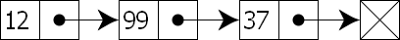Figure: singly-linked list containing two values: the value of the current node and a link to the next node

Here are a set of tests for our Linked List. The toString method for our LinkedList returns a String composed of a Lisp symbolic expression left parenthesis, list arguments and a right parenthesis.
`    public static void main(String [] args) {        System.out.println("Running linked list test");                final LinkedList list = new LinkedList("+");        list.assertEquals("" + list, "(+)");                // Start off backwards; insert using insertHead.        list.insertHead(new Node("1", null));               list.insertHead(new Node("2", null));        list.insertHead(new Node("3", null));        list.assertEquals("" + list, "(3 2 1 +)");        list.testStatus();        list.assertEquals(                "(3 2 1 +)",                list.getHead().stringifyNode());                final Node node = new Node("+", new Node("1", null));        list.assertEquals(                "(+ 1)",                node.stringifyNode());                final Node node2 = new Node("+", new Node("1", new Node("2", null)));        list.assertEquals(                "(+ 1 2)",                node2.stringifyNode());                final Node node3 = new Node("+", new Node("1", new Node("2", null)));        final LinkedList list2 = new LinkedList(node3);        list.assertEquals(                "(+ 1 2)",                list2.getHead().stringifyNode());        list.assertEquals(                "(+ 1 2)", "" + list2);                                             final LinkedList list3 = new LinkedList("+");        list3.assertEquals("" + list3, "(+)");        list3.insertTail(new Node("1", null));              list3.insertTail(new Node("2", null));        list3.insertTail(new Node("3", null));        list.assertEquals("" + list3, "(+ 1 2 3)");             list.testStatus();                        final LinkedList list4 = new LinkedList("1");               list4.insertTail(new Node("2", null));              list4.insertTail(new Node("3", null));        list4.insertTail(new Node("4", null));        list.assertEquals("" + list4, "(1 2 3 4)");        list.assertEquals("" + list4.numCompute('+'), "10.0");        list.assertEquals("" + list4.numCompute('*'), "24.0");    }`

Ding, Ding, Ding, *DING*

You may have noticed that I left out some implementation details on some of the methods used in the test case. They aren't totally relevant, use the source download at the bottom of the page to get all of the source. NumCompute is a relevant method and leads us into our Lisp implementation in Java. Essentially, the numCompute method is a traversal method to sum all of the Nodes in the list chain. This is kind of important, Lisp is a List processing language. Our traversal method is an operation on a singly linked list. I hope the light-bulb is going off for you.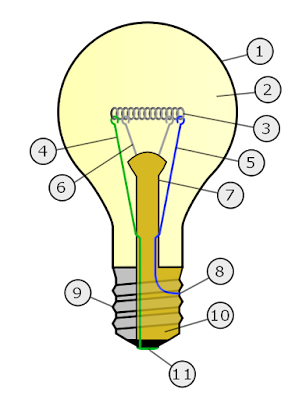`    /**     * Perform an arithmetic operation on the List.     * @param operation     * @return     */    public double numCompute(final char operation) {        double result = 0.0;        if (operation == '+' || operation == '-') {            result = 0.0;        } else if (operation == '*' || operation == '/') {            result = 1.0;        } else {            throw new RuntimeException("Invalid Operation: try +, -, *, /");        }        for (Node node = this.getHead(); node != null; node = node.getNext()) {            Double d = new Double((String) node.getData());            double x = d.doubleValue();            switch (operation) {                        case '+':                result += x;                break;            case '-':                result -= x;                break;            case '*':                result *= x;                break;            case '/':                result /= x;                break;            default:                throw new RuntimeException("Invalid Operation: try +, -, *, /");                            }        }                       return result;    }    `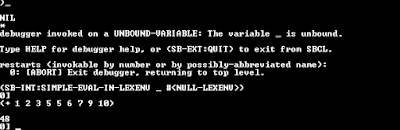Figure: SBCL prompt with the Lisp call (+ 1 2 3 5 5 6 7 9 10)

``The lisp code:(+ 1 2 3 4)``

Is essentially a representation of the following Java code:
`final LinkedList list4 = new LinkedList("1");       list4.insertTail(new Node("2", null));      list4.insertTail(new Node("3", null));list4.insertTail(new Node("4", null));list4.numCompute('+')`

On Lisp"On LISP's approximate 21st anniversary, no doubt something could be said about coming of age, but it seems doubtful that the normal life expectancy of a programming language is three score and ten. In fact, LISP seems to be the second oldest surviving programming language after Fortran, so maybe we should plan on holding one of these newspaper interviews in which grandpa is asked to what he attributes having lived to 100." -- John McCarthy

I don't want to delve too much into Lisp history or even the pros and cons; essentially Lisp has a rich history and is a very simple, powerful language. Lisp programs are represented by symbolic expressions as a simple linked list structure that is stored memory. We can determine the lisp function by the first ATOM in a list and perform that operation on the rest of the arguments. And there are a small set of selector and constructor operations expressed as functions (car, cdr and cons).

Lisp in Java, Mini JSchemeFigure: Picture of Peter Norvig, inventor of JScheme

Peter Norvig created JScheme, a Lisp Scheme dialect written in Java. It was created in 1998 and it has since been forked, extended and used quite extensively in other modern projects for the web and elsewhere. I mention JScheme because the code I am about present is all based on the JScheme interpreter. The code is copyrighted to Peter Norvig (including the picture of him above), I have only made modifications to make our Lisp even lighter than it was originally. Norvig's most recent implementation of JScheme contains 1905 lines of Java code. My implementation contains 905 lines wrapped in additional Javadoc comments.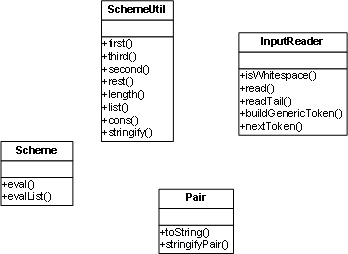There are eight classes in this smaller implementation. The core classes are shown in the UML class diagram. The InputReader is the most interesting class; if you are interested in learning about basic character/token parsing routines, you should look at this code. The read method is the first core method invoked. It is needed to read each character from the InputStream (E.g. an implementation of a FileInputStream) and return the tokens to the eval method.
`    public Object read() {        try {            Object token = nextToken();            if (token == "(") {                return readTail();            } else if (token == ")") {                System.out.println("WARN: Extra ')' ignored.");                return read();            } else {                return token;            } // End of the if - else        } catch (IOException e) {            System.out.println("WARN: On input, exception: " + e);            return EOF;        } // End try - catch    }    private Object readTail() throws IOException {        Object token = nextToken();        System.out.println("trace: readTail(): " + token);        if (token == EOF) {            final String msg = "ERROR: readTail() - EOF during read.";            System.err.println(msg);            throw (new RuntimeException(msg));        } else if (token == ")") {            return null;        } else if (token == ".") {            Object result = read();            token = nextToken();            if (token != ")") {                System.out.println("WARN: Missing ')'? Received " + token + " after .");            }            return result;        } else {            tokenStack.push(token);            return SchemeUtil.cons(read(), readTail());        }    }    private Object nextToken() throws IOException {        int ch;        // See if we should re-use a pushed char or token        // Task 1: Pop the token and character stacks        if (!this.tokenStack.empty() && (this.tokenStack.peek() != null)) {            return this.tokenStack.pop();        } else if (!this.charStack.empty() && (this.charStack.peek() != null)) {            ch = ((Character) this.charStack.pop()).charValue();        } else {            ch = inputReader.read();        }        // Ignore whitespace        // Task 2: Check for and ignore whitespace        while (isWhitespace((char) ch)) {            ch = inputReader.read();        }        System.out.println("trace: nextToken() -> " + (char) ch + " \$" + ch);        // See what kind of non-white character we got        // Task 3: Check if the character is of various token types.        switch (ch) {            case -1:                return EOF;            case TOK_LEFT_PAREN:                return "(";            case TOK_RIGHT_PAREN:                return ")";            ...            ...            default:                buff.setLength(0);                              int c = ch;                buildGenericToken(ch);                // Try potential numbers, but catch any format errors.                if (c == '.' || c == '+' || c == '-' || (c >= '0' && c <= '9')) {                    try {                        // Number type is currently in the buffer queue                        return new Double(buff.toString());                    } catch (NumberFormatException e) {                        ;                    }                } // End of If                return buff.toString().toLowerCase();        } // End of the Switch    }`

In that cluster of code, this small snippet should stick out in your mind:
`readTail:tokenStack.push(token);return SchemeUtil.cons(read(), readTail());`

Invocation of our cons operator. Cons, short for the term constructor, returns a new Pair object based on some data Node and possibly some pointer to another Pair. Notice that I am interchanging the term Pair and Node; where Node is the class in our LinkedList implementation. Essentially, a Pair is a Node and most of our Scheme processing is some variation of the LinkedList operations.
`    /**     * cons(x, y) is the same as new Pair(x, y).     *      * Cons presents and interesting function that is fundamental to lisp.     * Here are some examples of cons usage (tested in common lisp).     * <code>     * (cons 1 2):     * Pair pair = SchemeUtil.cons("1", "2");     * assertEquals("" + pair, "(1 . 2)");     *      * (cons 1 nil):     * Pair pair = SchemeUtil.cons("1", null);     * assertEquals("" + pair, "(1)");     *      * (cons 1 (cons 2 nil)):     *      * Pair pair = SchemeUtil.cons("1", SchemeUtil.cons("2", null));     * assertEquals("" + pair, "(1 2)");     *       * </code>     */    public static Pair cons(Object a, Object b) {        return new Pair(a, b);    }////// WHAT IS NEW PAIR????////** * A Pair has two fields, first and rest (or car and cdr). * This forms the basis of a simple, singly-linked list structure  * whose contents can be manipulated with cons, car, and cdr.  *  * "One mathematical consideration that influenced LISP was to  * express programs as applicative expressions built up  * from variables and constants using functions" -- John McCarthy * http://www-formal.stanford.edu/jmc/history/lisp/node3.html */public class Pair {    /**     * The first element of the pair.     */    private Object first;    /**     *  The other element of the pair.     */    private Object rest;    /** Build a pair from two components. * */    public Pair(Object first, Object rest) {        this.first = first;        this.rest = rest;    }    /**     * Return a String representation of the pair.     */    public String toString() {        return SchemeUtil.stringify(this);    }    /**     * Build up a String representation of the Pair in a StringBuffer.     */    void stringifyPair(StringBuffer buf) {        buf.append('(');        SchemeUtil.stringify(first, buf);        Object tail = rest;        while (tail instanceof Pair) {            buf.append(' ');            SchemeUtil.stringify(((Pair) tail).first, buf);            tail = ((Pair) tail).rest;        }        if (tail != null) {            buf.append(" . ");            SchemeUtil.stringify(tail, buf);        }        buf.append(')');    }}`

The input reader is our IO class for reading from the Stream and returning a PAIR to to the calling method. Once we have a Pair object (essentially a LinkedList structure), we can then perform Lisp operations on the given list elements. For example, here are two Test Case methods:
`public void testInputReaderPair() {        ByteArrayInputStream stream =             new ByteArrayInputStream("(+ 1 2 3)".getBytes());        InputReader reader = new InputReader(stream);        Object o = reader.read();        Pair p = (Pair) o;        assertEquals("(+ 1.0 2.0 3.0)", "" + p);    }    public void testEval1() {        Environment globalEnvironment = new Environment();        BuiltInFunction.installBuiltInFunctions(globalEnvironment);        ByteArrayInputStream stream = new ByteArrayInputStream("(+ 1 2 3)".getBytes());        InputReader reader = new InputReader(stream);        Object o = reader.read();        Scheme scheme = new Scheme();        Object res = scheme.eval(o, globalEnvironment);        double d = ((Double) res).doubleValue();        assertEquals(d, 6.0d, 0.01d);    }`

How do we get to performing Lisp operations on Lists?

In the testInputReaderPair test case, we convert a String representation of Lisp code and then create an InputReader object. Invoke the read method to return a Pair object. In this case, Pair is the head node in the LinkedList. The data in this Node is the String representation of the PLUS/ADD/+ operation. The rest of the elements are arguments to the function. The testEval1 test adds one more call to our first test. Eval. The Pair class is in memory, now we actually have to invoke Lisp operations to get any work done. In my example, I want to add those numbers to together to return a sum. The first element in the list is the function, the rest are my arguments.

"Based on the "token" and the parse rule being matched, the parser does something -- push the token on the parse stack, pop the previous token and emit some code, implement some "Semantic Action" based on the tokens and rules matched so far, etc. etc" -- SaveTheHubble (CrazyOnTap.com)

The Scheme.eval method is a driver method where the execution on the Pair begins.
`    public Object eval(Object x, Environment env) {         while (true) {            if (x instanceof String) {                          // VARIABLE                System.out.println("trace: eval - instance of String: " + x);                // Look up a variable or a procedure (built in function).                return env.lookup((String) x);                          } else if (!(x instanceof Pair)) {                 // CONSTANT                System.out.println("trace: eval - instance of Pair =>" + x);                return x;            } else {                // Procedure Call                System.out.println("trace: eval[t1] - instance of procedure call");                Object fn = SchemeUtil.first(x);                Object args = SchemeUtil.rest(x);                System.out.println("trace: eval[t2] - fn => [" + fn + "] args => " + args);                fn = eval(fn, env);                // Coerce the object to a procedure (E.g. '+/add' procedure)                Procedure p = Procedure.proc(fn);                               return p.apply(this, evalList(args, env));                                              } // End of if - else        } // End of While    } // End of Method`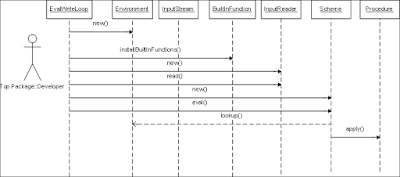The environment class is essentially a Hashtable data-structure for storing variable definitions. The key represents the name of the function and the value is an instance of the BuiltInFunction class. Other user defined variables are also stored here (this feature is not implemented in MiniJScheme). There is a major variation from Norvig's JScheme implemention of the Environment class. He used a LinkedList structure to store all of the variables. To lookup a variable by name, you traverse the list until the variable/function is found. I used simple Hashtable get and put operations. It is the least interesting of all of the classes, but probably the most important. If you don't have a reference to the ADD operation, then you can't a sum on a set of numbers.
`public class Environment {        private Map mapDataVars = new HashMap();        public Environment() {    }    /**      * Find the value of a symbol, in this environment or a parent.      */    public Object lookup(String symbol) {        Object o = this.mapDataVars.get(symbol);        if (o != null) {            return o;        } else {            return SchemeUtil.error("Unbound variable: [" + symbol + "]");        }    }    /** Add a new variable,value pair to this environment. */    public Object define(Object var, Object val) {                this.mapDataVars.put(var, val);                 if (val instanceof Procedure                && ((Procedure) val).getName().equals(Procedure.DEFAULT_NAME)) {            ((Procedure) val).setName(var.toString());        }        return var;    }       public Environment defineBuiltInProc(String name, int id, int minArgs) {        define(name, new BuiltInFunction(id, minArgs, minArgs));        return this;    }   }`

We are finally at the point to talk about calling Lisp functions. Here are some basic "Scheme" operations. The cons/constructor(new Pair) operation should be pretty straightforward. Same for the "first" function. I didn't include the code for numCompute because the implementation is the same as the LinkedList code shown earlier.

`public static Environment installBuiltInFunctions(Environment env)  {        int n = Integer.MAX_VALUE;        env                 .defineBuiltInProc("cons",     CONS,      2)                         .defineBuiltInProc("*",        TIMES,     0, n)                  .defineBuiltInProc("+",        PLUS,      0, n)         .defineBuiltInProc("-",        MINUS,     1, n)         .defineBuiltInProc("/",        DIVIDE,    1, n)                 .defineBuiltInProc("caaaar",         CXR,       1)         .defineBuiltInProc("caar",           CXR,       1)               .defineBuiltInProc("cadr",         SECOND,      1)                   .defineBuiltInProc("cdar",           CXR,       1)         .defineBuiltInProc("cddr",           CXR,       1)                  .defineBuiltInProc("car",            CAR,       1)         .defineBuiltInProc("cdr",            CDR,       1)                                  .defineBuiltInProc("*",        TIMES,     0, n)                  .defineBuiltInProc("+",        PLUS,      0, n)         .defineBuiltInProc("-",        MINUS,     1, n)         .defineBuiltInProc("/",        DIVIDE,    1, n);        return env;    }   /**      * Apply a primitive built-in-function to a list of arguments.     */    public Object apply(Scheme interp, Object args) {        // First make sure there are the right number of arguments.         int nArgs = SchemeUtil.length(args);        if (nArgs < minArgs) {            return SchemeUtil.error("too few args, " + nArgs + ", for " + this.getName() + ": "                    + args);        } else if (nArgs > maxArgs) {            return SchemeUtil.error("too many args, " + nArgs + ", for " + this.getName()                    + ": " + args);        } // End of the If        Object x = SchemeUtil.first(args);        Object y = SchemeUtil.second(args);        switch (idNumber) {            case PLUS:                return numCompute(args, '+', 0.0);            case MINUS:                return numCompute(SchemeUtil.rest(args), '-', num(x));            case TIMES:                return numCompute(args, '*', 1.0);            case DIVIDE:                return numCompute(SchemeUtil.rest(args), '/', num(x));                      case THIRD:                return SchemeUtil.third(x);            case CONS:                return SchemeUtil.cons(x, y);            case CAR:                return SchemeUtil.first(x);            case CDR:                return SchemeUtil.rest(x);            case CXR:                for (int i = this.getName().length() - 2; i >= 1; i--) {                    x = (this.getName().charAt(i) == 'a') ? SchemeUtil.first(x) : SchemeUtil.rest(x);                }                return x;            default:                return SchemeUtil.error("internal error: unknown primitive: " + this                        + " applied to " + args);        }    } // End of the Apply`

More Test Cases

Here are some more Java test cases and their Scheme/Common Lisp equivalent:

`public class TestPairList extends TestCase {        public static final String test1 = "(cons 1 2)";    public static final String test2 = "(cons 1 (cons 2 ()))";        public void testBasicBuildPair() {        // Construct a linked list/pair of        // a function '+', 1 and 2        // (+ 1 2)        Pair pair = new Pair("+", new Pair("1", new Pair("2", null)));        assertEquals("" + pair, "(+ 1 2)");    }       public void testPair1() {        // Construct a linked list/pair of        // a function '+', 1 and 2        // (+ 1 2)        Pair pair = new Pair("+", new Pair("1", new Pair("2", ":test")));               assertEquals("" + pair, "(+ 1 2 . :test)");    }       /**     * (cons 1 2).       */    public void testCons() {        Pair pair = SchemeUtil.cons("1", "2");        assertEquals("" + pair, "(1 . 2)");    }    /**     * (cons 1 nil).     */    public void testConsOne() {        Pair pair = SchemeUtil.cons("1", null);        assertEquals("" + pair, "(1)");    }    /**     * (cons 1 (cons 2 nil)).         */    public void testConsTwo() {        Pair pair = SchemeUtil.cons("1", SchemeUtil.cons("2", null));        assertEquals("" + pair, "(1 2)");    }        public void testEvalCons1() {        Environment globalEnvironment = new Environment();        BuiltInFunction.installBuiltInFunctions(globalEnvironment);        ByteArrayInputStream stream = new ByteArrayInputStream(test1.getBytes());        InputReader reader = new InputReader(stream);        Object o = reader.read();        Scheme scheme = new Scheme();        Object res = scheme.eval(o, globalEnvironment);        System.out.println(res);    }    public void testEvalCons2() {        Environment globalEnvironment = new Environment();        BuiltInFunction.installBuiltInFunctions(globalEnvironment);        ByteArrayInputStream stream = new ByteArrayInputStream(test2.getBytes());        InputReader reader = new InputReader(stream);        Object o = reader.read();        Scheme scheme = new Scheme();        Object res = scheme.eval(o, globalEnvironment);        assertEquals("" + res, "(1.0 2.0)");    }}`

Conclusion

This concludes my run down of a Scheme/Lisp implementation in Java starting from Linked List data structures and continuing to the interpreter code. There is a lot that can be added to Norvig's implementation. Add full support for a R5RS Scheme Implementation. Add better input/output support. Copy interesting functions from Arc or Common Lisp. Write a Scheme compiler. In fact, the source code in my implementation and Norvig's is fairly straightforward, I was planning on writing a Forth interpreter based on some of this code. Lisp's defining data-structure is the List. Forth's is the stack. It won't be nearly as complete as the Factor programming language, but it would still be a fun project.

Source Code

References

http://norvig.com/jscheme.html
http://factorcode.org/
http://www-formal.stanford.edu/jmc/recursive/node3.html
http://www-formal.stanford.edu/jmc/

Addendum: Implementation of Closures, introducing lambda

I did not add Closures to my implementation of JScheme, but it can be added in twenty lines of code. Here is Norvig's implementation of the Closure class:

"A closure is a user-defined procedure. It is "closed" over the environment in which it was created. To apply the procedure, bind the parameters to the passed in variables, and evaluate the body."
`public class Closure extends Procedure {    Object parms;    Object body;    Environment env;        /** Make a closure from a parameter list, body, and environment. **/    public Closure (Object parms, Object body, Environment env) {        this.parms = parms;    this.env = env;    this.body = (body instanceof Pair && rest(body) == null)        ? first(body) : cons("begin", body);    }    /**     * Apply a closure to a list of arguments.     */    public Object apply(Scheme interpreter, Object args) {        return interpreter.eval(body, new Environment(parms, args, env));    }}`

The Scheme.eval method; the lambda List closure is a block/list of Lisp code that contains a set of operations that are defined without a name (anonymous function call). In common lisp, we would do the following:
`Break 1 > (defun abc (x) (funcall #'(lambda (y) (+ x y)) 5))ABCBreak 1 > (abc 4)9Break 1 >`
`/**  * Evaluate an object, x, in an environment.  */public Object eval(Object x, Environment env) {  Object fn = first(x);  Object args = rest(x);   if (fn == "define") { // DEFINE      if (first(args) instanceof Pair)          return env.define(first(first(args)), eval(cons(                                "lambda", cons(rest(first(args)),                                                rest(args))),                                env));      else          return env.define(first(args), eval(second(args), env));  } else if (fn == "lambda") { // LAMBDA    return new Closure(first(args), rest(args), env);  }`